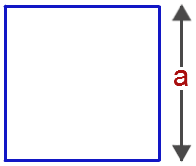# Perimeter of a Square Formula

The total distance outside the square is the perimeter of the square. We know that a square has 4 sides and the perimeter of the sum of the length of all the 4 sides. All the sides of a square are equal and hence the perimeter is taken as four times the one side.Perimeter of a Square Formula is given as,

$$\begin{array}{l}\large Perimeter\;of\;a\;Square(P)=4a\end{array}$$

Where,
a = Side of the square

### Solved Example

Question: Find the perimeter of a square whose side is 16 cm.

Solution:

Given,
Side of the square = a = 16 cm

Perimeter of a Square = 4a
= 4 x 16
= 64 cm